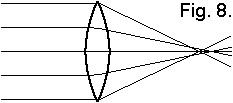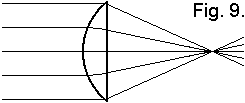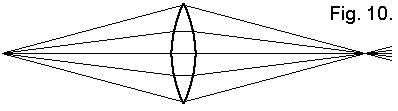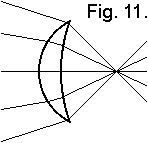# Narrow-bandwidth Television Association## Chapter 21

### Spherical aberration

One defect that affects correct focusing of lenses is called spherical aberration. This is caused by a different focus point of the centre part and the edges of the lens, see fig. 8.This spherical aberration can be minimised by curving the lens, i.e. moving the edge in respect to the centre part of the lens. In the case of fig. 8 you might see that we have to move the edges to the right. We now obtain a lens with one spherical surface (convex) and one almost flat surface (planar).The amount of the spherical aberration depends on the way light is entering and leaving the lens. When light is a parallel beam at one side of the lens, then the minimal spherical aberration occurs when the other surface is flat. Between the two lenses of a condenser system, see fig. 4, the beam is parallel so the condenser system has to be flat on both sides. To be precise, in fig. 4 the left lens should have been drawn less curved, thus thinner than the lens on the right.When the beam enters the lens in a diverging way and leaves in a converging way, both surfaces should be convex. For reasons of symmetry both surfaces must have the same curvature if object and image have the same distance from the lens, see fig. 10.When light enters the lens in a converging way and is even more focused by the lens, then we need a convex-concave lens. In practice, this is done in the better photo cameras, to further minimise spherical aberration, by using several lenses instead of just one.
Spectacles always have convex-concave lenses, so they are not at all optimised for spherical aberration. It looks as if this is only done to prevent your lashes touching the glass.
As a general conclusion: lenses should be used in such a way that both glass surfaces contribute more or less the same amount of focusing action. The bend in the rays of light should be almost equal on both sides of the lens.